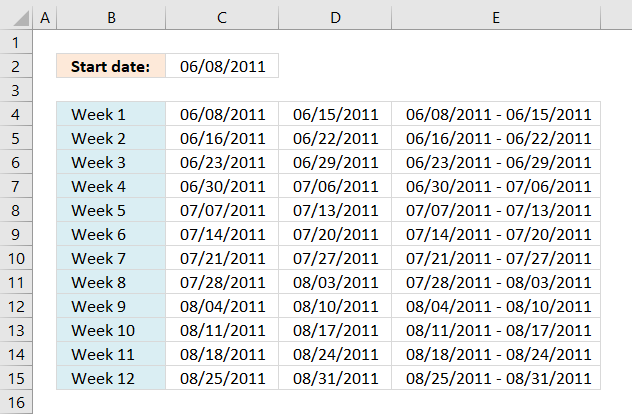Author: Oscar Cronquist Article last updated on January 22, 2019Shannon asks:I need a formula that if I enter a start date in field B1 such as 6/8/11 it will give me the date ranges for 7 days in fields B3-B14. Does that make sense?

Basically I want a formula that will tell me when a client is admitted to services on 6/8/11, their week 1 is 6/8/11 to 6/15/11; week 2 is 6/16/11-6/22/11 etc through 12 weeks.

I want the initial date in B1 to be the only value that I have to change to produce these results. Is that possible?

I could create a big formula in cell range B3:B14 but instead, I am going to simplify formulas and use three columns.

The formulas are dynamic meaning when a date is entered in cell B1 cell range B3:D14 is instantly recalculated.

Formula in cell B3:

=B1

Formula in cell C3:

=\$C\$2+ROWS(\$A\$1:A1)*7

Copy (Ctrl + c) cell C3 and paste (Ctrl + v) to cell range C4:C14.

Formula in cell D3:

=TEXT(B3, "M/D/YY")&" - "&TEXT(C3, "M/D/YY")

Copy (Ctrl + c) cell D3 and paste (Ctrl + v) to cell range D4:D14.

Formula in cell B4:

=C3+1

Copy (Ctrl + c) cell B4 and paste (Ctrl + v) to cell range B5:B14.

### Explaining formula in cell C3

=\$B\$1+ROW(A1)*7

#### Step 1 - Create an absolute cell reference to start date

=\$B\$1+ROWS(\$A\$1:A1)*7

\$B\$1 is an absolute cell reference. To create a reference to another cell, double click cell C3. Type = and left click cell B1. The formula becomes =B1.

Let's convert the cell reference to an absolute cell reference. Absolute cell references are cell references that don't change when the cell is copied and pasted to another cell. Press F4.

The formula becomes: =\$B\$1.

#### Step 2 - Make dates with interval

=\$B\$1+ROW(A1)*7

ROW(A1). A1 is a relative cell reference to cell A1. A relative cell reference is a cell reference that adjusts and change when copied.  ROW(A1) returns the row number of a reference. ROW(A1) returns 1.

ROW(A1)*7 becomes

1*7

and returns 7.

#### Step 3 - All together

=\$B\$1+ROW(A1)*7

becomes

40702+1*7

and returns 40709. Formatted as a date, cell C3 returns 6/15/11.

Cell C4 becomes  =\$B\$1+ROW(A2)*7

=40702+2*7

becomes

=40702+14

and returns 40716. Formatted as a date, cell C4 returns 6/22/11.